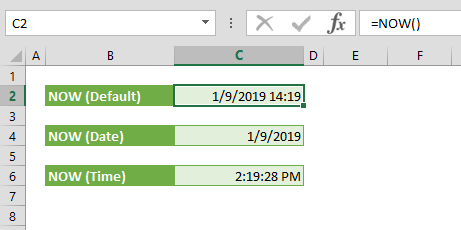The NOW function returns the serial number of the current date and time. The return value gets updated with every recalculation. In this guide, we’re going to show you how to use the NOW function and also throw in some tips and error handling methods.

• All versions

NOW()

# Arguments

• No arguments

Examples

=NOW()
formula returns the current date-time value of your local machine. If the cell's number format is General, Excel automatically applies a Custom number format according to your localization settings.# Tips

• Excel stores dates as serial numbers. Excel assumes the date 1/1/1900 as 1 and increases this number by 1 for each day. For example; 12/31/2018 is equal to 43465. Because of this situation, avoid to use dates as text although the DAY function can operate with dates match with your local format. Use DATE function to generate Date
• Use number format options to display only specific part of a date-time value. For example;
• Use plus (+) and minus (-) operators to add/remove days to current time: How to use day information from date data in Excel
• There is a VBA function with same name and same functionality:
`Dim mydate as Date`

mydate = Now

` `